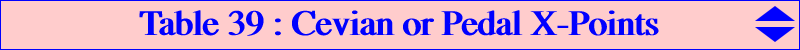This page is dedicated to the memory of Steve Sigur who left us on July, 5 2008.   Let X be a center in the plane of the reference triangle ABC. Let P be a point with cevian or pedal triangle PaPbPc. We say that P is a cevian X-point or pedal X-point if the triangles ABC and PaPbPc share this same center X. See the Hyacinthos message #16699 by Antreas Hatzipolakis. For example, there is only one cevian G-point which is G itself : the centroid of the cevian triangle of G is G. Similarly, there is also only one pedal G-point which is O : the centroid of the pedal triangle of O is G. In both cases, the triangle is the medial triangle. It seems out of reach to investigate the most general situation and we will only examinate a few examples involving the most common triangles centers. Any contribution is welcome.Pedal O-points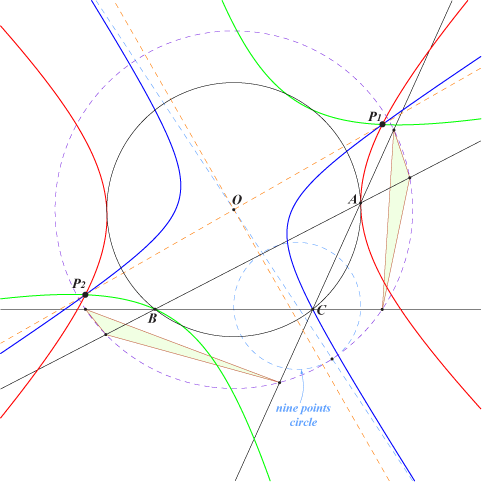In Hyacinthos #16700, Jean-Pierre Ehrmann observes that one can find two pedal O-points namely the real foci P1, P2 of the inscribed conic with center O. These are isogonal conjugate points on the McCay cubic K003. The common pedal circle of P1 and P2 has radius (R+OH)/2 which should mean that this circle touches the nine points circle on the Euler line [end quote]. We may consider that the two other (imaginary) foci are also pedal O-points. Indeed, when we express that the perpendicular bisector of PbPc passes through O, we obtain that P must lie on a rectangular hyperbola with center O, passing through A (red curve). Similarly, we obtain two other rectangular hyperbolas and these meet at the four required foci.Cevian H-pointsThere are three – one at least real – cevian H-points lying on the Euler line. These are the isogonal conjugates of the common points (apart the Lemoine point) of the Jerabek hyperbola and the diagonal conic passing through X(6), X(31), X(48), X(154), X(1613), X(2578), X(2579) and the vertices of the tangential triangle T. Recall that X(154) is the centroid of T thus the construction is easy. The first figure shows a configuration with the isogonal conjugates of three real points P1, P2, P3. The second figure shows one of the corresponding triangles.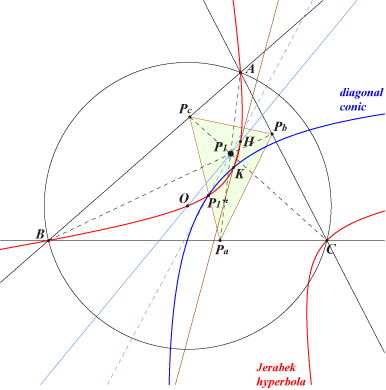Pedal H-pointsWhen we express that the Pa-altitude of PaPbPc passes through H, we obtain that P must lie on a conic passing through A. Two other similar conics are defined and this shows that there are four pedal H-points. These lie on the rectangular hyperbola passing through X(3), X(4), X(1498), X(2574), X(2575)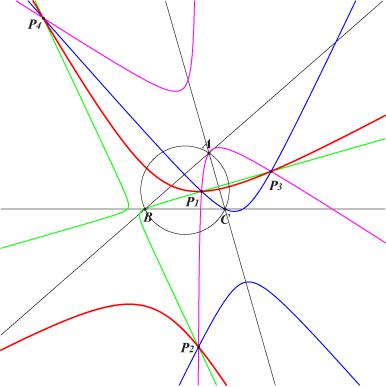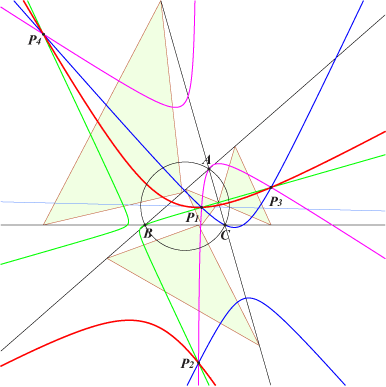Cevian O-points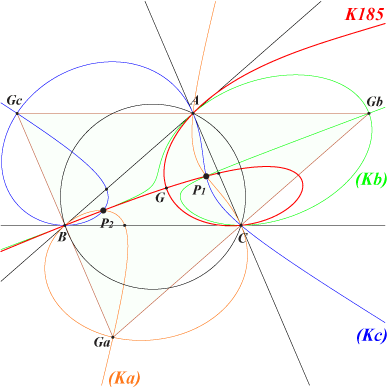When we express that the perpendicular bisector of PbPc passes through O, we obtain that P must lie on a nodal isotomic circumcubic (Ka) with node Ga, vertex of the antimedial triangle. (Ka) is tangent at B to BA, at C to CA. It meets BC again at the A-vertex of the cevian triangle of X(76). The tangent at A is the A-symmedian. The cubics (Kb) and (Kc) are defined similarly. These three cubics belong to a same pencil hence there are six cevian O-points which are two by two isotomic conjugates and obviously cyclocevian conjugates. The pencil is formed by isotomic nKs with root on the de Longchamps line i.e. the trilinear polar of X(76) or the isotomic transform of the circumcircle. It contains one and only one K0 which is the Hirst-Kiepert cubic K185.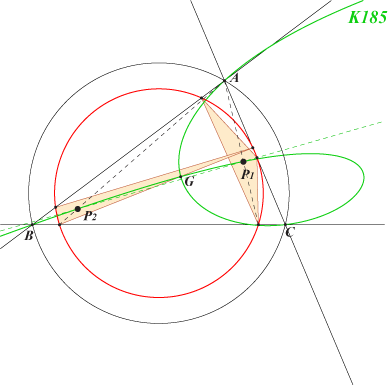The figure shows these points P1, P2 with their common cevian circle.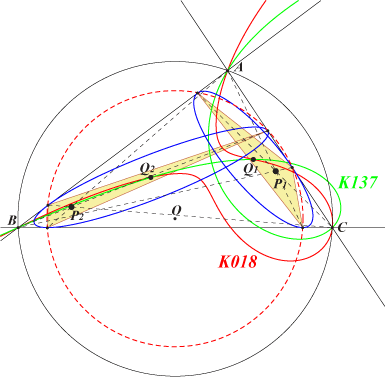The cevian O-points are also the perspectors of the inconics whose centers are the common points of all the isogonal nK0s with root at infinity. These cubics form a pencil containing K018, K137, K325. Recall that these cubics are the loci of M such that M and its isogonal conjugate are conjugated with respect to a circumconic passing through G. The figure shows two such centers Q1, Q2 and P1, P2, the isotomic conjugates of their anticomplements.Pedal N-points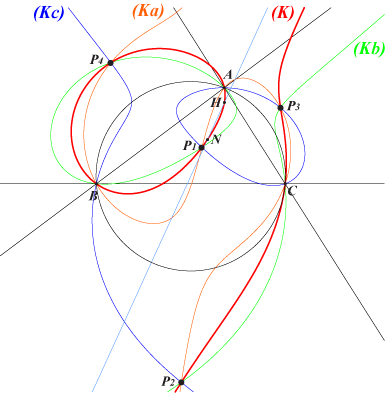The nine point center of the pedal triangle of P coincide with the nine point center N of ABC if and only if P lies on three circular circumcubics (Ka), (Kb), (Kc) belonging to a same pencil of cubics. Hence, there are four pedal N-points P1, P2, P3, P4. This pencil contains (K) the only cubic of the pencil passing through N. This also contains H, X(30), X(1141).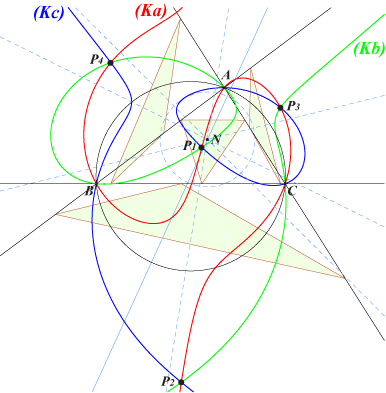The figure shows the four corresponding pedal triangles with their Euler lines concurring at N.Cevian N-points